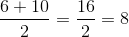## Example Questions

### Example Question #21 : Factors / Multiples

The number 9 is the second smallest integer with 3 factors, 1, 3, and 9. What is the sum of the factors of the smallest integer with only 3 factors?

17
7
13
4
15
Explanation:

Here we must do two things. First we must find the smallest integer with 3 factors, then we must add those factors so that we can obtain our answer.

Looking at numbers less than 9 with only 3 factors, the only possibility is the number 4, whose factors are 1, 2, and 4.

The sum of these factors is 1 + 2 + 4 = 7

### Example Question #22 : Factors / Multiples

If a and b are both factors of 64, which of the following could be a * b?

128

200

1920

34

128

Explanation:

The factors of 64 are: 1,2,4,8,16,32,64. Therefore, 128 could be the product of 16 and 8.

### Example Question #23 : Factors / Multiples

Which of the following is not a factor of 52?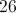Explanation:

Listing all the factors of 52: 1,2,4,13,26,52.

3 is not one of the factors.

### Example Question #24 : Factors / Multiples

Which of the following lists all the factors of 36?

1, 36

1, 2, 3, 4, 6, 9, 12, 18, 36

2, 3

2, 4, 12, 18, 36

36, 72

1, 2, 3, 4, 6, 9, 12, 18, 36

Explanation:

1, 2, 3, 4, 6, 9, 12, 18, 36 are all of the factors of 36.

### Example Question #25 : Factors / Multiples

What are the factors of the number 12?

2, 6

2, 3, 6

1, 2, 3, 4, 6, 12

1, 12

3, 4

1, 2, 3, 4, 6, 12

Explanation:

The factors of a number are all the numbers that can be multiplied by an integer to get that number.

### Example Question #26 : Factors / Multiples

What is the sum of the greatest common factor (GCF) and the least common multiple (LCM) of,, and?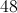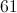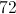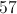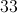Explanation: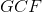:  the largest factor that divides evenly into all numbers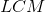:  the smallest non-zero number that divides evenly into all numbers.  If you are unable to find the GCF, then it is 1.

Prime factorize all numbers: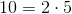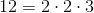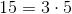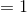, because all numbers are relatively prime.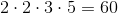Therefore,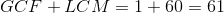.

### Example Question #27 : Factors / Multiples

What is the power of the greatest prime factor of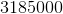?4Explanation:

To get the answer to this question, just carefully prime factor the value:

First,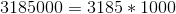Now,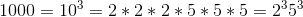For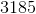, begin by dividing by: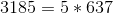Now, this is a bit trickier for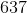. This happens to be divisible by: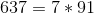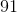also is divisible by: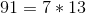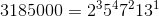### Example Question #28 : Factors / Multiples

How many factors are there for the number?Explanation:

To find the number of factors of a given number, the easiest thing to do is to make a table of the factors, starting withand that number. So, for, we get: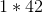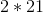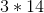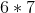At this point, things begin to repeat. Thus, the total number of factors is.

### Example Question #1 : How To Factor A Number

What is the median value of the factors of?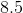There is no median.Explanation:

To find the number of factors of a given number, the easiest thing to do is to make a table of the factors, starting withand that number. So, for, we get: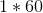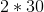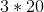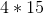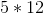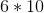At this point, the values begin to repeat. This means that there are an even number of factors. At the "middle" of the list, we findand. To find the median of the list, you merely need to take the average of these two numbers: